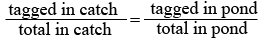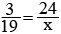SEARCH HOMEMath Central Quandaries & QueriesQuestion from Peter, a student: At the beginning of a study 24 fish are caught, tagged, and released back into a large pond containing fish. A few days later, 19 fish are caught from the pond and three of them have tags. Using this ratio, how many total fish would you expect to be living in the pond? The answer is 152. Why is that?Hi Peter,

Lets let x represent the total population of fish in the pond.
The ratio of tagged fish to the total fish population is therefore 24 : x

Assuming this ratio of "tagged to total" will be found for whatever number of fish get caught would mean that the ratio of "tagged to total" in the catch is the same as the ratio of "tagged to total" for the pond. We can solve this type of problem with a proportion:Substituting in the numbers we get:Solving proportions such as these involves cross-multiplication

3x = (19)(24) After dividing both sides by 3 to isolate x we see that

x = 152

Hope this helps,
LeeanneMath Central is supported by the University of Regina and The Pacific Institute for the Mathematical Sciences.